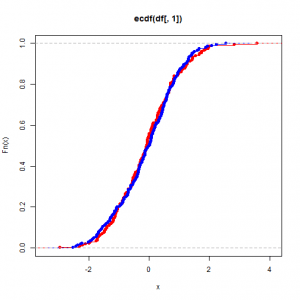# Hacking statistics or: How I Learned to Stop Worrying About Calculus and Love Stats Exercises (Part-1)

July 1, 2017
By

Want to share your content on R-bloggers? click here if you have a blog, or here if you don't.Statistics are often taught in school by and for people who like Mathematics. As a consequence, in those class emphasis is put on leaning equations, solving calculus problems and creating mathematics models instead of building an intuition for probabilistic problems. But, if you read this, you know a bit of R programming and have access to a computer that is really good at computing stuff! So let’s learn how we can tackle useful statistic problems by writing simple R query and how to think in probabilistic terms.

This exercise set will introduce you to common distributions and simple sampling related concepts which will be useful when we’ll see more advance concept like bootstrapping and A/B testing in some future post in this series.

Answers to the exercises are available here.

Exercise 1
Use `rnorm()` to generate 100 points in the interval [0,1], then plot those points in an histogram.

Exercise 2
Repeat exercise 1, but this time with, 500, 1000 and 10000 points.

Exercise 3
We can see that the more points are generated, the more the histogram become symmetric and centered around 0. The reason for this is that `rnorm()` generate the point based on a function which dictate precisely what should be the proportion of points in each subinterval of [0,1] and that function has for characteristics to be symmetric, centered around 0 with two inflection points which make his shape look like a bell. That density function is called a Normal distribution and a lot of practical application use it.

Use the `dnorm()` function to plot the density function of a normal distribution of mean 0 and standard deviation of 1 and add it to the last histogram you plot.

The histograms we plotted before where discrete approximation of this continuous function. Since we deal with a random process, each bin of the histogram doesn’t fill up with the correct frequency evenly. As a consequence, it can take a lot of observation before the histogram represent the underling distribution of the random process. Here lies the biggest problem that statisticians face: can we make decisions based on a sample of size n or does a bigger sample would reveal that the random process is distributed under another density function.
Exercise 4
We can use this shape to verify if a random process is a normal process. Another useful plot is the empirical cumulative distribution function (ECDF) which represent visually the probability that an observation is smaller than a certain value. Plot the cumulative histogram of 10000 points from a standard normal distribution, then add the ECDF curve to the plot by using the `pnorm()` function.

Exercise 5
There’s a lot of distribution other than the standard normal distribution that you can find in practice. To familiarize with the shape of those function, plot the density function of those common functions:

• Exponential with a rate of 0.5
• Exponential with a rate of 1
• Exponential with a rate of 2
• Exponential with a rate of 10
• Gamma with a shape of 1 and a scale equal to 2
• Gamma with a shape of 2 and a scale equal to 2
• Gamma with a shape of 5 and a scale equal to 2
• Gamma with a shape of 5 and a scale equal to 0.5
• Student with 10 degree of freedom
• Student with 5 degree of freedom
• Student with 2 degree of freedom
• Student with 1 degree of freedom

Exercise 6
Repeat the steps of exercise 5, but plot the ECDF instead.

Exercise 7
Now it’s time to put what we learn to test! Download this dataset and try to find if those observations have the same distribution. Start by looking at the histogram of both variables in this dataset.

Exercise 8
Both dataset seems symmetric and to have the same domain.

Exercise 9
Use the `ecdf()` function to plot the empirical cumulative distribution function of both sample.

Exercise 10
The plots indicate that there’s little difference between the distribution of both sample. Using the Kolmogorov-Smirnov test is a good way to determine if two sample share the same distribution. This test measure the maximum difference between the ecdf of both samples and compute the probability of such difference to appear when the ecdf are the same.

Use the `ks.test()` function to run the Kolmogorov-Smirnov test on both samples.

The first sample in the dataset was sampled from a Student distribution with 10 degrees of freedom, while the second was sampled from a standart normal distribution. Both density functions are quite similar and in practice using one over the other won’t make a huge difference, but some function have a heavy tail, meaning that they can create some rare events who take huge value. Those events usually won’t appear in a small sample and failing to differentiate such function for other can generate huge estimation errors. In the next post, we’ll see method to distinguish between two similar distributions.

R-bloggers.com offers daily e-mail updates about R news and tutorials about learning R and many other topics. Click here if you're looking to post or find an R/data-science job.
Want to share your content on R-bloggers? click here if you have a blog, or here if you don't.